# Level Modifier

• Create a cross table Profit per Product Hierarchy by Customer Country.
• Add a new calculated measure Segment % that calculates each Product/Customer Country combination as a percentage of the grand total profit.
• Instead of referring to all columns or all rows on a certain level it will often be relevant to refer to a single column or row at the 'All' level:

sum(0, 0, m1) / sum(all, all, m1) -> sum(0, 0, m1) / sum(all, d-1(l0), m1)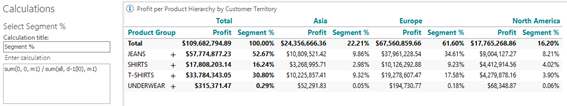Another example of the level parameter being useful can be observed in the simple trend calculation, where the level parameter can make a calculation robust in case of hierarchy expansion.

• Add a crosstab – Profit per Product Hierarchy (Product Group) and by Time Hierarchy (Year).
• Calculate a difference between the last 2 columns:
Trend = sum(d-1, 0, m1) – sum(d-2, 0, m1)

This calculation always calculates the 2 last columns – if the hierarchy is expanded the calculation will calculate the 2 last columns at the expanded level – as shown below:

Not expanded: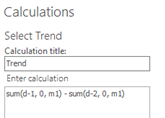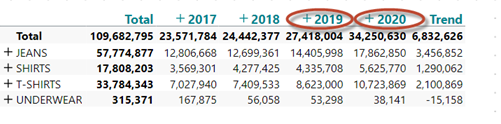Partially expanded (now the Trend calculation calculates the difference between Q4 and Q3 in 2015):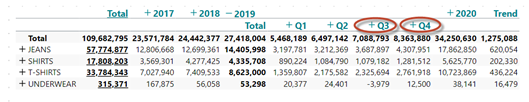This could be the intention – and in that case there is no problem, but if you want to make the calculation robust to expansion of the hierarchy you can use the level parameter.

Trend = sum(d-1(l1), 0, m1) – sum(d-2(l1), 0, m1)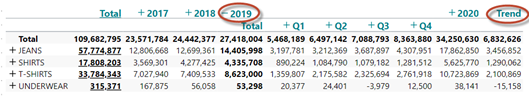As level 1 in this case specifies the year level the calculation is now robust to expansion.